Created July 31, 2021

# AI Enhanced Security Camera

Provide secure building entry by using microwave sensor and DepthAI camera.

124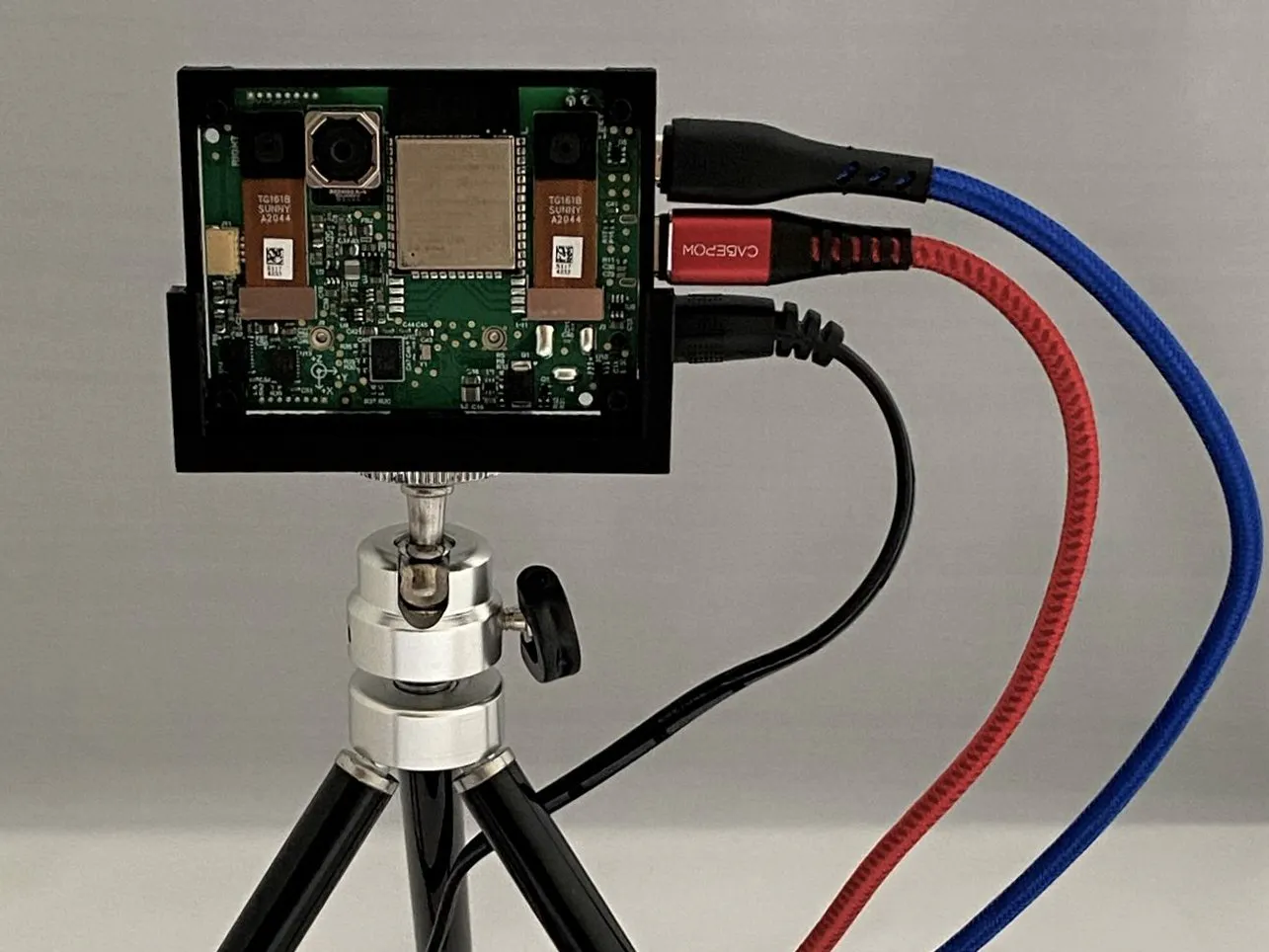## Things used in this project

### Hardware components

 LUX-ESP32
×1
 Microwave Proximity Detector RCWL-0516
×1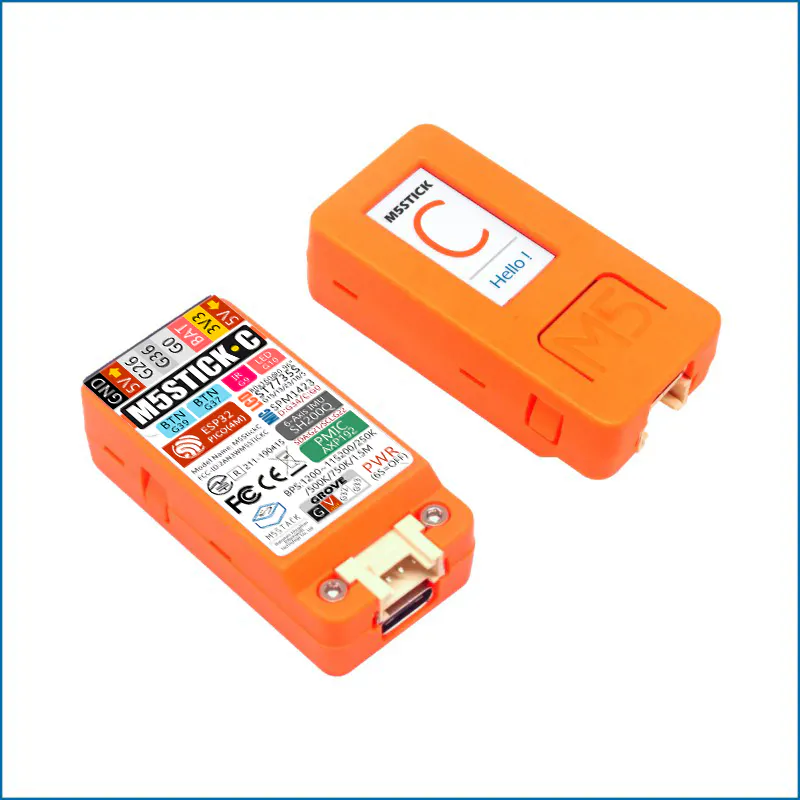M5Stack M5StickC ESP32-PICO Mini IoT Development Board
×1
 M5Stack M5StickC 18650C
×1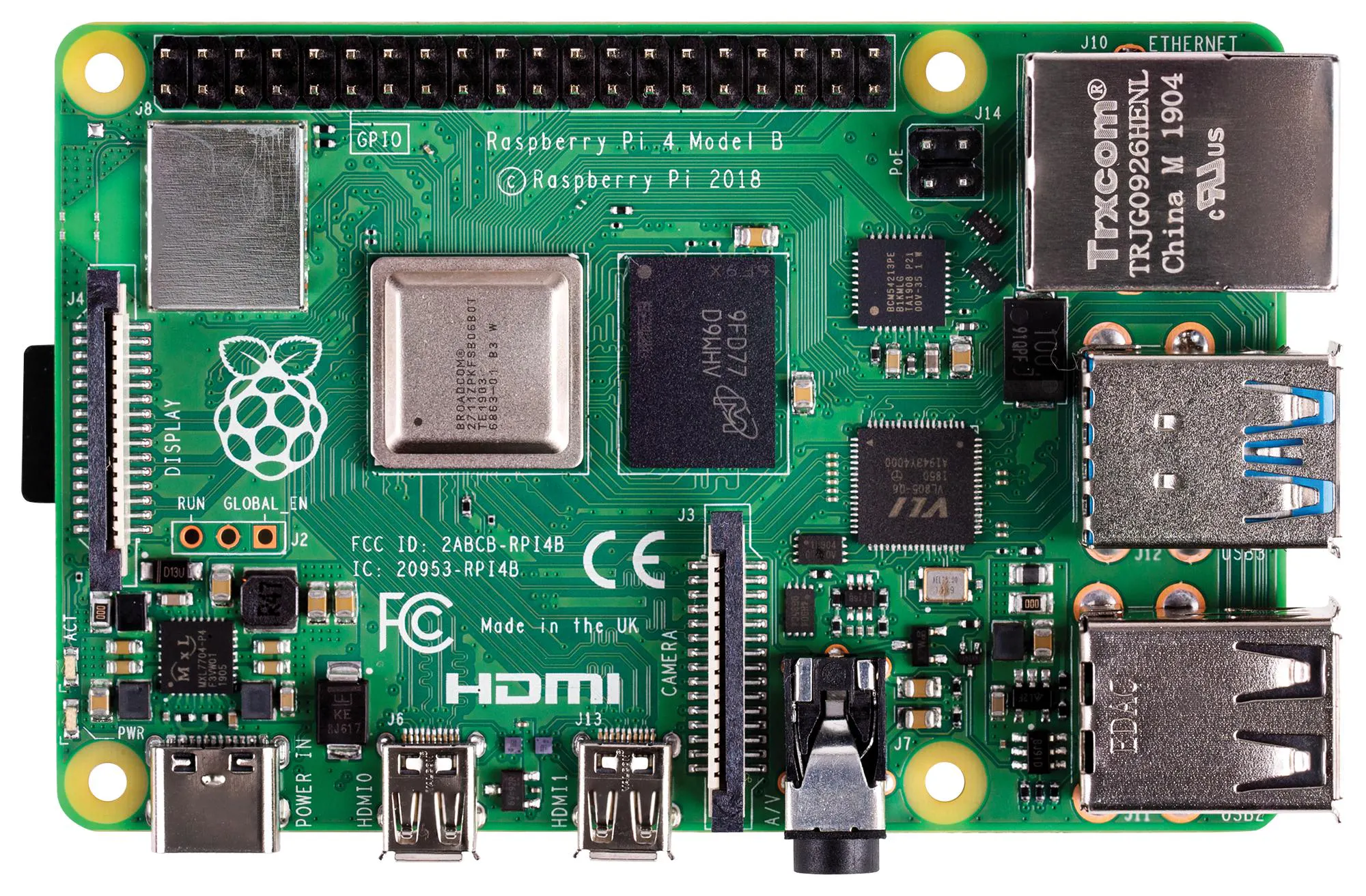Raspberry Pi 4 Model B
×1

### Software apps and online services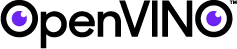Intel OpenVINO toolkit
 Luxonis DepthAI Gen2 API
 Espressif ESP-IDFArduino IDE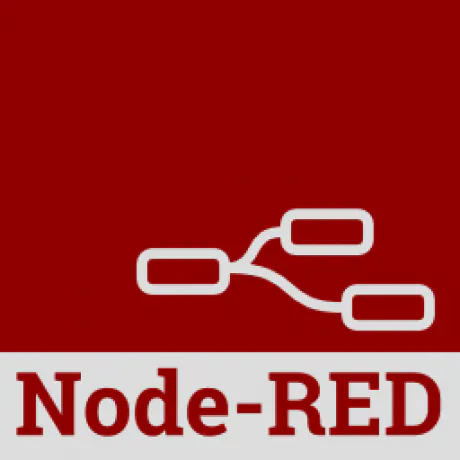Node-RED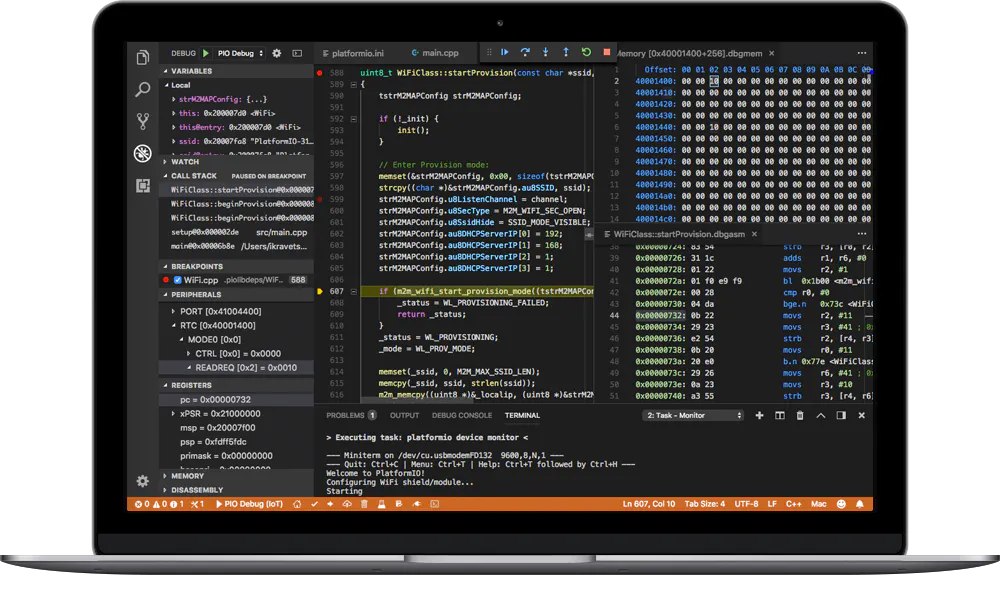PlatformIO IDE

### Hand tools and fabrication machines

 AnyCubic Mega S 3D Printer

## Schematics

### Portable Microwave Sensor Schematic

RCWL-0516 Sensor on M5StickC with 18650 Battery HAT

## Code

### M5StickC_RCWL_0516_MQTT_Static

Arduino
Publishes Microwave detector status via MQTT
```/**
* M5StickC_RCWL_0156_MQTT.ino
*
*  Created on: 08.03.2021
*
* the arduino_secrets.h file:
* #define SECRET_SSID ""    // network name
* #define SECRET_PASS ""    // network password
* #define SECRET_MQTT_USER "public" // broker username
* #define SECRET_MQTT_PASS "public" // broker password
*
*
*/

#include <Arduino.h>
#include <WiFi.h>
#include <PubSubClient.h>
#include "arduino_secrets.h"
#include <M5StickC.h>
#include <Wire.h>
#include "AXP192.h"

#define USE_SERIAL Serial

// LED Pin
const int ledPin = 10;

//const char* mqtt_server = "192.168.1.144";
const char* mqtt_server = "10.0.0.234";

IPAddress primaryDNS(8, 8, 8, 8);   //optional
IPAddress secondaryDNS(8, 8, 4, 4); //optional

WiFiClient espClient;
PubSubClient client(espClient);
long lastMsg = 0;
char msg;
int value = 0;
int detectionCount = 0;
int newDetection = 0;
int statusChange = 0;

void IRAM_ATTR Ext_INT1_ISR()
{
statusChange = 1;
// Turn on LED if motion detected
detectionCount++;
}

void setup() {

M5.begin();
M5.Lcd.setRotation(3);
M5.Lcd.fillScreen(BLACK);
M5.Lcd.setCursor(10, 10, 2);
M5.Lcd.println("Microwave Sensor");
pinMode(M5_BUTTON_HOME, INPUT);
pinMode(26, INPUT);
pinMode(26, INPUT_PULLDOWN);
attachInterrupt(26, Ext_INT1_ISR, CHANGE);  // RISING  FALLING  HIGH  LOW  CHANGE

USE_SERIAL.begin(115200);
//    while(!USE_SERIAL);

USE_SERIAL.println();
USE_SERIAL.println();
USE_SERIAL.println();

setup_wifi();
client.setServer(mqtt_server, 1883);
client.setCallback(callback);

pinMode(ledPin, OUTPUT);
digitalWrite(ledPin, HIGH);

}

void setup_wifi() {
delay(10);
// We start by connecting to a WiFi network
Serial.println();
Serial.print("Connecting to ");
//  Serial.println(ssid);
Serial.println(SECRET_SSID);

if(!WiFi.config(local_IP, gateway, subnet, primaryDNS, secondaryDNS)) {
Serial.println("Static IP Failed to configure");
}

WiFi.begin(SECRET_SSID, SECRET_PASS);

while (WiFi.status() != WL_CONNECTED) {
delay(500);
Serial.print(".");
}

Serial.println("");
Serial.println("WiFi connected");
Serial.println(WiFi.localIP());
}

void callback(char* topic, byte* message, unsigned int length) {
Serial.print("Message arrived on topic: ");
Serial.print(topic);
Serial.print(". Message: ");
String messageTemp;

for (int i = 0; i < length; i++) {
Serial.print((char)message[i]);
messageTemp += (char)message[i];
}
Serial.println();

// Feel free to add more if statements to control more GPIOs with MQTT

// If a message is received on the topic floor2/output, you check if the message is either "on" or "off".
// Changes the output state according to the message
if (String(topic) == "rcwl/led") {
Serial.print("Changing led to ");
if(messageTemp == "on"){
Serial.println("on");
digitalWrite(ledPin, LOW);
}
else if(messageTemp == "off"){
Serial.println("off");
digitalWrite(ledPin, HIGH);
}
}
}

void reconnect() {
// Loop until we're reconnected
while (!client.connected()) {
Serial.print("Attempting MQTT connection...");
// Attempt to connect
if (client.connect("ESP32Client2")) {
Serial.println("connected");
// Subscribe
client.subscribe("rcwl/led");
} else {
Serial.print("failed, rc=");
Serial.print(client.state());
Serial.println(" try again in 5 seconds");
// Wait 5 seconds before retrying
delay(5000);
}
}
}

void loop() {
if (!client.connected()) {
reconnect();
}
client.loop();

if (statusChange) {
// Publish status
char statusBuf;
char dopplerBuf;
String statusString;
String dopplerString;
if (newDetection){
statusString = "Motion";
dopplerString = "1";
newDetection = 0;
} else {
statusString = "Secure";
dopplerString = "0";
}
statusString.toCharArray(statusBuf,8);
client.publish("rcwl/status",statusBuf);
dopplerString.toCharArray(dopplerBuf,8);
client.publish("rcwl/doppler",dopplerBuf);
statusChange = 0;
}

long now = millis();
if (now - lastMsg > 60000) {
lastMsg = now;

// Convert the value to a char array
char batString;
dtostrf(M5.Axp.GetBatVoltage(), 1, 2, batString);
Serial.print("Battery: ");
Serial.println(batString);
client.publish("rcwl/battery", batString);
Serial.print("Detection Count: ");
Serial.println(detectionCount);
detectionCount = 0;
}

delay(1000);
}
```

### gen2-face-recognition_10fps_main.py

Python
gen2-face-recognition code modified to limit camera inferencing input to 10 FPS
```# coding=utf-8
import os
from pathlib import Path
from queue import Queue
import argparse
from time import monotonic

import cv2
import depthai
import numpy as np
from imutils.video import FPS

parser = argparse.ArgumentParser()
"-nd", "--no-debug", action="store_true", help="prevent debug output"
)
"-cam",
"--camera",
action="store_true",
help="Use DepthAI 4K RGB camera for inference (conflicts with -vid)",
)

"-vid",
"--video",
type=str,
help="The path of the video file used for inference (conflicts with -cam)",
)

"-db",
"--databases",
action="store_true",
help="Save data (only used when running recognition network)",
)

"-n",
"--name",
type=str,
default="",
help="Data name (used with -db) [Optional]",
)

args = parser.parse_args()

debug = not args.no_debug

is_db = args.databases

if args.camera and args.video:
raise ValueError(
'Command line parameter error! "-Cam" cannot be used together with "-vid"!'
)
elif args.camera is False and args.video is None:
raise ValueError(
'Missing inference source! Use "-cam" to run on DepthAI cameras, or use "-vid <path>" to run on video files'
)

def to_planar(arr: np.ndarray, shape: tuple):
return cv2.resize(arr, shape).transpose((2, 0, 1)).flatten()

def to_nn_result(nn_data):
return np.array(nn_data.getFirstLayerFp16())

def run_nn(x_in, x_out, in_dict):
nn_data = depthai.NNData()
for key in in_dict:
nn_data.setLayer(key, in_dict[key])
x_in.send(nn_data)
return x_out.tryGet()

def frame_norm(frame, *xy_vals):
return (
np.clip(np.array(xy_vals), 0, 1) * np.array(frame * (len(xy_vals) // 2))[::-1]
).astype(int)

def correction(frame, angle=None, invert=False):
h, w = frame.shape[:2]
center = (w // 2, h // 2)
mat = cv2.getRotationMatrix2D(center, angle, 1)
affine = cv2.invertAffineTransform(mat).astype("float32")
corr = cv2.warpAffine(
frame,
mat,
(w, h),
flags=cv2.INTER_CUBIC,
borderMode=cv2.BORDER_CONSTANT,
)
if invert:
return corr, affine
return corr

def cosine_distance(a, b):
if a.shape != b.shape:
raise RuntimeError("array {} shape not match {}".format(a.shape, b.shape))
a_norm = np.linalg.norm(a)
b_norm = np.linalg.norm(b)
similarity = np.dot(a, b.T) / (a_norm * b_norm)

return similarity

databases = "databases"

if not os.path.exists(databases):
os.mkdir(databases)

def create_db(face_fame, results):
name = args.name
font = cv2.FONT_HERSHEY_PLAIN
font_scale = 1
font_color = (0, 255, 0)
line_type = 1
if len(name) == 0:
while 1:
cc = face_fame.copy()
cv2.putText(
cc,
f"Who is he/she？\r\n{name}",
(0, 30),
font,
font_scale,
font_color,
line_type,
)
cv2.imshow("who?", cc)

k = cv2.waitKey(0)
if k == 27:  # Esc
# == ord("q"):
cv2.destroyAllWindows()
raise StopIteration()
# name = input("Who is he/she ? (length >2 )")
if k == 13:  # Enter
if len(name) > 0:
save = True
break
else:
cv2.putText(
cc,
"Did not enter a name? Enter it again" f"{name}",
(0, 30),
font,
font_scale,
font_color,
line_type,
)
cv2.imshow("who?", cc)
k = cv2.waitKey(0)
if k == 27:
break
continue
if k == 225:  # Shift
break
if k == 8:  # backspace
name = name[:-1]
continue
else:
name += chr(k)
continue
try:
db_ = [db[j] for j in db.files][:]
except Exception as e:
db_ = []
db_.append(np.array(results))
np.savez_compressed(f"{databases}/{name}", *db_)

for file in os.listdir(databases):
filename = os.path.splitext(file)
if filename == ".npz":
label = filename
db_dic = {}
for label in list(labels):
db_dic[label] = [db[j] for j in db.files]
return db_dic

class DepthAI:
def __init__(
self,
file=None,
camera=False,
):
self.file = file
self.camera = camera
self.fps_cam = FPS()
self.fps_nn = FPS()
self.create_pipeline()
self.start_pipeline()
self.fontScale = 1 if self.camera else 2
self.lineType = 0 if self.camera else 3

def create_pipeline(self):
print("Creating pipeline...")
self.pipeline = depthai.Pipeline()

if self.camera:
# ColorCamera
print("Creating Color Camera...")
self.cam = self.pipeline.createColorCamera()
self.cam.setPreviewSize(self._cam_size, self._cam_size)
self.cam.setResolution(
depthai.ColorCameraProperties.SensorResolution.THE_4_K
)
self.cam.setInterleaved(False)
self.cam.setBoardSocket(depthai.CameraBoardSocket.RGB)
self.cam.setColorOrder(depthai.ColorCameraProperties.ColorOrder.BGR)
self.cam.setFps(10.0)

self.cam_xout.setStreamName("preview")

self.create_nns()

print("Pipeline created.")

def create_nns(self):
pass

def create_nn(self, model_path: str, model_name: str, first: bool = False):
"""

:param model_path: model path
:param model_name: model abbreviation
:param first: Is it the first model
:return:
"""
# NeuralNetwork
print(f"Creating {model_path} Neural Network...")
model_nn = self.pipeline.createNeuralNetwork()
model_nn.setBlobPath(str(Path(f"{model_path}").resolve().absolute()))
model_nn.input.setBlocking(False)
if first and self.camera:
else:
model_in.setStreamName(f"{model_name}_in")

model_nn_xout.setStreamName(f"{model_name}_nn")

def create_mobilenet_nn(
self,
model_path: str,
model_name: str,
conf: float = 0.5,
first: bool = False,
):
"""

:param model_path: model name
:param model_name: model abbreviation
:param conf: confidence threshold
:param first: Is it the first model
:return:
"""
# NeuralNetwork
print(f"Creating {model_path} Neural Network...")
model_nn = self.pipeline.createMobileNetDetectionNetwork()
model_nn.setBlobPath(str(Path(f"{model_path}").resolve().absolute()))
model_nn.setConfidenceThreshold(conf)
model_nn.input.setBlocking(False)

if first and self.camera:
else:
model_in.setStreamName(f"{model_name}_in")

model_nn_xout.setStreamName(f"{model_name}_nn")

def start_pipeline(self):
self.device = depthai.Device(self.pipeline)
print("Starting pipeline...")

self.start_nns()

if self.camera:
self.preview = self.device.getOutputQueue(
name="preview", maxSize=4, blocking=False
)

def start_nns(self):
pass

def put_text(self, text, dot, color=(0, 0, 255), font_scale=None, line_type=None):
font_scale = font_scale if font_scale else self.fontScale
line_type = line_type if line_type else self.lineType
dot = tuple(dot[:2])
cv2.putText(
img=self.debug_frame,
text=text,
org=dot,
fontFace=cv2.FONT_HERSHEY_COMPLEX,
fontScale=font_scale,
color=color,
lineType=line_type,
)

def draw_bbox(self, bbox, color):
cv2.rectangle(
img=self.debug_frame,
pt1=(bbox, bbox),
pt2=(bbox, bbox),
color=color,
thickness=2,
)

def parse(self):
if debug:
self.debug_frame = self.frame.copy()

s = self.parse_fun()
# if s :
#     raise StopIteration()
if debug:
cv2.imshow(
"Camera_view",
self.debug_frame,
)
self.fps_cam.update()
if cv2.waitKey(1) == ord("q"):
cv2.destroyAllWindows()
self.fps_cam.stop()
self.fps_nn.stop()
print(
f"FPS_CAMERA: {self.fps_cam.fps():.2f} , FPS_NN: {self.fps_nn.fps():.2f}"
)
raise StopIteration()

def parse_fun(self):
pass

def run_video(self):
cap = cv2.VideoCapture(str(Path(self.file).resolve().absolute()))
while cap.isOpened():
break

try:
self.parse()
except StopIteration:
break

cap.release()

def run_camera(self):
while True:
in_rgb = self.preview.tryGet()
if in_rgb is not None:
shape = (3, in_rgb.getHeight(), in_rgb.getWidth())
self.frame = (
in_rgb.getData().reshape(shape).transpose(1, 2, 0).astype(np.uint8)
)
self.frame = np.ascontiguousarray(self.frame)
try:
self.parse()
except StopIteration:
break

@property
def cam_size(self):
return self._cam_size

@cam_size.setter
def cam_size(self, v):
self._cam_size = v

def run(self):
self.fps_cam.start()
self.fps_nn.start()
if self.file is not None:
self.run_video()
else:
self.run_camera()
del self.device

class Main(DepthAI):
def __init__(self, file=None, camera=False):
self.cam_size = (300, 300)
super(Main, self).__init__(file, camera)
self.face_frame_corr = Queue()
self.face_frame = Queue()
self.face_coords = Queue()
self.labels = set()
if not is_db:

def create_nns(self):
self.create_mobilenet_nn(
"models/face-detection-retail-0004_openvino_2021.2_4shave.blob",
"mfd",
first=self.camera,
)

self.create_nn(
)
self.create_nn(
"models/face-recognition-mobilefacenet-arcface_2021.2_4shave.blob",
"arcface",
)

def start_nns(self):
if not self.camera:
self.mfd_in = self.device.getInputQueue("mfd_in")
self.mfd_nn = self.device.getOutputQueue("mfd_nn", 4, False)
self.arcface_in = self.device.getInputQueue("arcface_in", 4, False)
self.arcface_nn = self.device.getOutputQueue("arcface_nn", 4, False)

def run_face_mn(self):
if not self.camera:
nn_data = run_nn(
self.mfd_in, self.mfd_nn, {"data": to_planar(self.frame, (300, 300))}
)
else:
nn_data = self.mfd_nn.tryGet()
if nn_data is None:
return False

bboxes = nn_data.detections
for bbox in bboxes:
face_coord = frame_norm(
self.frame.shape[:2], *[bbox.xmin, bbox.ymin, bbox.xmax, bbox.ymax]
)
self.face_frame.put(
self.frame[face_coord : face_coord, face_coord : face_coord]
)
self.face_coords.put(face_coord)
self.draw_bbox(face_coord, (10, 245, 10))
return True

while self.face_frame.qsize():
face_frame = self.face_frame.get()
nn_data = run_nn(
{"data": to_planar(face_frame, (60, 60))},
)
if nn_data is None:
return False

out = np.array(nn_data.getLayerFp16("angle_r_fc"))
self.face_frame_corr.put(correction(face_frame, -out))

return True

def run_arcface(self):
while self.face_frame_corr.qsize():
face_coords = self.face_coords.get()
face_fame = self.face_frame_corr.get()

nn_data = run_nn(
self.arcface_in,
self.arcface_nn,
{"data": to_planar(face_fame, (112, 112))},
)

if nn_data is None:
return False
self.fps_nn.update()
results = to_nn_result(nn_data)

if is_db:
create_db(face_fame, results)
else:
conf = []
max_ = 0
label_ = None
for label in list(self.labels):
for j in self.db_dic.get(label):
conf_ = cosine_distance(j, results)
if conf_ > max_:
max_ = conf_
label_ = label
conf.append((max_, label_))
name = conf if conf >= 0.5 else (1 - conf, "UNKNOWN")
self.put_text(
f"name:{name}",
(face_coords, face_coords - 35),
(0, 0, 255),0.9,1
)
self.put_text(
f"conf:{name * 100:.2f}%",
(face_coords, face_coords - 10),
(0, 0, 255),0.9,1
)
return True

def parse_fun(self):
if self.run_face_mn():
if self.run_arcface():
return True

if __name__ == "__main__":
if args.video:
Main(file=args.video).run()
else:
Main(camera=args.camera).run()
```

### Swap_Tiles_using_Depth.py

Python
DepthAI Python program to switch left and right images in output tiles based on depth (distance from object)
```#!/usr/bin/env python3

import cv2
import depthai as dai

stepSize = 0.05

newConfig = False

# Create pipeline
pipeline = dai.Pipeline()

camRgb = pipeline.createColorCamera()
camRgb.setPreviewSize(1000, 500)
camRgb.setInterleaved(False)
maxFrameSize = camRgb.getPreviewHeight() * camRgb.getPreviewHeight() * 3

# In this example we use 2 imageManips for splitting the original 1000x500
# preview frame into 2 500x500 frames
manip1 = pipeline.createImageManip()
manip1.initialConfig.setCropRect(0, 0, 0.5, 1)
manip1.setMaxOutputFrameSize(maxFrameSize)

manip2 = pipeline.createImageManip()
manip2.initialConfig.setCropRect(0.5, 0, 1, 1)
manip2.setMaxOutputFrameSize(maxFrameSize)

xout1.setStreamName('out1')

xout2.setStreamName('out2')

# Script node
script = pipeline.create(dai.node.Script)
script.inputs['Spatial_Data'].setBlocking(False)
script.inputs['Manip1_Out'].setBlocking(False)
script.inputs['Manip2_Out'].setBlocking(False)
script.setScript("""
while True:
slc_in = node.io['Spatial_Data'].get()
img1_in = node.io['Manip1_Out'].get()
img2_in = node.io['Manip2_Out'].get()
spatialData = slc_in.getSpatialLocations()
for depthData in spatialData:
depthMin = depthData.depthMin
depthMax = depthData.depthMax
depthAvg = depthData.depthAverage
if depthAvg < 2000 :
node.io['Xout1_In'].send(img1_in)
node.io['Xout2_in'].send(img2_in)
else :
node.io['Xout1_In'].send(img2_in)
node.io['Xout2_in'].send(img1_in)
node.warn(f"Hello from script {depthAvg}")
""")

# Define sources and outputs
monoLeft = pipeline.createMonoCamera()
monoRight = pipeline.createMonoCamera()
stereo = pipeline.createStereoDepth()
spatialLocationCalculator = pipeline.createSpatialLocationCalculator()

xoutDepth.setStreamName("depth")
xoutSpatialData.setStreamName("spatialData")
xinSpatialCalcConfig.setStreamName("spatialCalcConfig")

# Properties
monoLeft.setResolution(dai.MonoCameraProperties.SensorResolution.THE_400_P)
monoLeft.setBoardSocket(dai.CameraBoardSocket.LEFT)
monoRight.setResolution(dai.MonoCameraProperties.SensorResolution.THE_400_P)
monoRight.setBoardSocket(dai.CameraBoardSocket.RIGHT)

lrcheck = False
subpixel = False

stereo.initialConfig.setConfidenceThreshold(255)
stereo.setLeftRightCheck(lrcheck)
stereo.setSubpixel(subpixel)

# Config
topLeft = dai.Point2f(0.4, 0.4)
bottomRight = dai.Point2f(0.6, 0.6)

config = dai.SpatialLocationCalculatorConfigData()
config.depthThresholds.lowerThreshold = 100
config.depthThresholds.upperThreshold = 10000
config.roi = dai.Rect(topLeft, bottomRight)

spatialLocationCalculator.setWaitForConfigInput(False)

# Connect to device and start pipeline
with dai.Device(pipeline) as device:

# Output queue will be used to get the depth frames from the outputs defined above
depthQueue = device.getOutputQueue(name="depth", maxSize=4, blocking=False)
spatialCalcQueue = device.getOutputQueue(name="spatialData", maxSize=4, blocking=False)
spatialCalcConfigInQueue = device.getInputQueue("spatialCalcConfig")
q1 = device.getOutputQueue(name="out1", maxSize=4, blocking=False)
q2 = device.getOutputQueue(name="out2", maxSize=4, blocking=False)

color = (255, 255, 255)

print("Use WASD keys to move ROI!")

while True:
inDepth = depthQueue.get() # Blocking call, will wait until a new data has arrived
in1 = q1.tryGet()
in2 = q2.tryGet()

depthFrame = inDepth.getFrame()
depthFrameColor = cv2.normalize(depthFrame, None, 255, 0, cv2.NORM_INF, cv2.CV_8UC1)
depthFrameColor = cv2.equalizeHist(depthFrameColor)
depthFrameColor = cv2.applyColorMap(depthFrameColor, cv2.COLORMAP_HOT)

spatialData = spatialCalcQueue.get().getSpatialLocations()
for depthData in spatialData:
roi = depthData.config.roi
roi = roi.denormalize(width=depthFrameColor.shape, height=depthFrameColor.shape)
xmin = int(roi.topLeft().x)
ymin = int(roi.topLeft().y)
xmax = int(roi.bottomRight().x)
ymax = int(roi.bottomRight().y)

depthMin = depthData.depthMin
depthMax = depthData.depthMax

fontType = cv2.FONT_HERSHEY_TRIPLEX
cv2.rectangle(depthFrameColor, (xmin, ymin), (xmax, ymax), color, cv2.FONT_HERSHEY_SCRIPT_SIMPLEX)
cv2.putText(depthFrameColor, f"X: {int(depthData.spatialCoordinates.x)} mm", (xmin + 10, ymin + 20), fontType, 0.5, 255)
cv2.putText(depthFrameColor, f"Y: {int(depthData.spatialCoordinates.y)} mm", (xmin + 10, ymin + 35), fontType, 0.5, 255)
cv2.putText(depthFrameColor, f"Z: {int(depthData.spatialCoordinates.z)} mm", (xmin + 10, ymin + 50), fontType, 0.5, 255)
# Show the frame
cv2.imshow("depth", depthFrameColor)
if in1 is not None:
cv2.imshow("Tile 1", in1.getCvFrame())

if in2 is not None:
cv2.imshow("Tile 2", in2.getCvFrame())

key = cv2.waitKey(1)
if key == ord('q'):
break
elif key == ord('w'):
if topLeft.y - stepSize >= 0:
topLeft.y -= stepSize
bottomRight.y -= stepSize
newConfig = True
elif key == ord('a'):
if topLeft.x - stepSize >= 0:
topLeft.x -= stepSize
bottomRight.x -= stepSize
newConfig = True
elif key == ord('s'):
if bottomRight.y + stepSize <= 1:
topLeft.y += stepSize
bottomRight.y += stepSize
newConfig = True
elif key == ord('d'):
if bottomRight.x + stepSize <= 1:
topLeft.x += stepSize
bottomRight.x += stepSize
newConfig = True

if newConfig:
config.roi = dai.Rect(topLeft, bottomRight)
cfg = dai.SpatialLocationCalculatorConfig()
spatialCalcConfigInQueue.send(cfg)
newConfig = False
```

### Swap_NNs_on_Proximity.py

Python
DepthAI Python program to switch between Object Detection and Face Detection NNs based on depth (distance from object)
```# first, import all necessary modules
from pathlib import Path

import blobconverter
import cv2
import depthai as dai
import numpy as np

stepSize = 0.05
newConfig = False

# MobilenetSSD label texts
labelMap = ["background", "face", "bicycle", "bird", "boat", "bottle", "bus", "car", "cat", "chair", "cow",
"diningtable", "dog", "horse", "motorbike", "person", "pottedplant", "sheep", "sofa", "train", "tvmonitor"]

# Create pipeline
pipeline = dai.Pipeline()

# Set up Color Camera as input
camRgb = pipeline.createColorCamera()
camRgb.setPreviewSize(300, 300)
camRgb.setInterleaved(False)

# Set up Object Detection NN
obj_detection_nn = pipeline.createMobileNetDetectionNetwork()
# We're using a blobconverter tool to retreive the MobileNetSSD blob automatically from OpenVINO Model Zoo
obj_detection_nn.setBlobPath(str(blobconverter.from_zoo(name='mobilenet-ssd', shaves=6)))
# Next, we filter out the detections that are below a confidence threshold. Confidence can be anywhere between <0..1>
obj_detection_nn.setConfidenceThreshold(0.5)
# Next, we link the camera 'preview' output to the neural network detection input, so that it can produce detections

# Set up Face Detection NN
face_detection_nn = pipeline.createMobileNetDetectionNetwork()
# We're using a blobconverter tool to retreive the MobileNetSSD blob automatically from OpenVINO Model Zoo
face_detection_nn.setBlobPath(str(blobconverter.from_zoo(name='face-detection-retail-0004', shaves=6)))
# Next, we filter out the detections that are below a confidence threshold. Confidence can be anywhere between <0..1>
face_detection_nn.setConfidenceThreshold(0.5)
# Next, we link the camera 'preview' output to the neural network detection input, so that it can produce detections

# Set up Camera preview link to host
xout_rgb.setStreamName("rgb")
# Linking camera preview to XLink input, so that the frames will be sent to host

# Set up NN link to host
xout_nn.setStreamName("nn")

# Script node
script = pipeline.create(dai.node.Script)
script.inputs['Spatial_Data'].setBlocking(False)
script.inputs['Obj_NN_Out'].setBlocking(False)
script.inputs['Face_NN_Out'].setBlocking(False)
script.setScript("""
while True:
slc_in = node.io['Spatial_Data'].get()
nn1_in = node.io['Obj_NN_Out'].get()
nn2_in = node.io['Face_NN_Out'].get()
spatialData = slc_in.getSpatialLocations()
for depthData in spatialData:
depthMin = depthData.depthMin
depthMax = depthData.depthMax
depthAvg = depthData.depthAverage
if depthAvg > 2000 :
node.io['Xout_NN_In'].send(nn1_in)
else :
node.io['Xout_NN_In'].send(nn2_in)
node.warn(f"Hello from script {depthAvg}")
""")

# Define sources and outputs
monoLeft = pipeline.createMonoCamera()
monoRight = pipeline.createMonoCamera()
stereo = pipeline.createStereoDepth()
spatialLocationCalculator = pipeline.createSpatialLocationCalculator()

# Properties
monoLeft.setResolution(dai.MonoCameraProperties.SensorResolution.THE_400_P)
monoLeft.setBoardSocket(dai.CameraBoardSocket.LEFT)
monoRight.setResolution(dai.MonoCameraProperties.SensorResolution.THE_400_P)
monoRight.setBoardSocket(dai.CameraBoardSocket.RIGHT)

lrcheck = False
subpixel = False

stereo.initialConfig.setConfidenceThreshold(255)
stereo.setLeftRightCheck(lrcheck)
stereo.setSubpixel(subpixel)

# Config
topLeft = dai.Point2f(0.3, 0.3)
bottomRight = dai.Point2f(0.7, 0.7)

config = dai.SpatialLocationCalculatorConfigData()
config.depthThresholds.lowerThreshold = 100
config.depthThresholds.upperThreshold = 10000
config.roi = dai.Rect(topLeft, bottomRight)

spatialLocationCalculator.setWaitForConfigInput(False)

# Connect to device and start pipeline
with dai.Device(pipeline) as device:

# Output queues will be used to get the frames from the outputs defined above
q_rgb = device.getOutputQueue("rgb")
q_nn = device.getOutputQueue("nn")

frame = None
detections = []

def frameNorm(frame, bbox):
normVals = np.full(len(bbox), frame.shape)
normVals[::2] = frame.shape
return (np.clip(np.array(bbox), 0, 1) * normVals).astype(int)

def displayFrame(name, frame):
color = (255, 0, 0)
for detection in detections:
bbox = frameNorm(frame, (detection.xmin, detection.ymin, detection.xmax, detection.ymax))
cv2.putText(frame, labelMap[detection.label], (bbox + 10, bbox + 20), cv2.FONT_HERSHEY_TRIPLEX, 0.5, color)
cv2.putText(frame, f"{int(detection.confidence * 100)}%", (bbox + 10, bbox + 40), cv2.FONT_HERSHEY_TRIPLEX, 0.5, color)
cv2.rectangle(frame, (bbox, bbox), (bbox, bbox), color, 2)
# Show the frame
cv2.imshow(name, frame)

while True:
#        inDepth = depthQueue.get() # Blocking call, will wait until a new data has arrived

in_rgb = q_rgb.tryGet()
in_nn = q_nn.tryGet()

if in_rgb is not None:
frame = in_rgb.getCvFrame()

if in_nn is not None:
detections = in_nn.detections

if frame is not None:
displayFrame("camera", frame)

key = cv2.waitKey(1)
if key == ord('q'):
break
q
```

### 4k-cam_main.py

Python
gen2-people-tracker code for use with 4K camera input (only minor changes to Luxonis depthai-experiments example)
```#!/usr/bin/env python3

import sys
from pathlib import Path

import blobconverter
import cv2
import depthai as dai
import numpy as np
import time
import argparse
from time import monotonic

# Labels
labelMap = ["background", "person" ]

# Get argument first
parser = argparse.ArgumentParser()
help="Minimum distance the person has to move (across the x/y axis) to be considered a real movement")
args = parser.parse_args()

parentDir = Path(__file__).parent

nnPath = args.nn or blobconverter.from_zoo(name="person-detection-retail-0013", shaves=7)

# Minimum distance the person has to move (across the x/y axis) to be considered a real movement
THRESH_DIST_DELTA = args.threshold

# Start defining a pipeline
pipeline = dai.Pipeline()

# Create and configure the detection network
detectionNetwork = pipeline.createMobileNetDetectionNetwork()
detectionNetwork.setBlobPath(str(Path(nnPath).resolve().absolute()))
detectionNetwork.setConfidenceThreshold(0.5)
detectionNetwork.input.setBlocking(False)

# Create and configure the color camera
colorCam = pipeline.createColorCamera()
colorCam.setPreviewSize(544, 320)
colorCam.setResolution(dai.ColorCameraProperties.SensorResolution.THE_4_K)
colorCam.setInterleaved(False)
colorCam.setColorOrder(dai.ColorCameraProperties.ColorOrder.BGR)
# Connect RGB preview to the detection network

# Create and configure the object tracker
objectTracker = pipeline.createObjectTracker()
objectTracker.setDetectionLabelsToTrack()  # Track people
# Possible tracking types: ZERO_TERM_COLOR_HISTOGRAM, ZERO_TERM_IMAGELESS
objectTracker.setTrackerType(dai.TrackerType.ZERO_TERM_COLOR_HISTOGRAM)
# Take the smallest ID when new object is tracked, possible options: SMALLEST_ID, UNIQUE_ID
objectTracker.setTrackerIdAssigmentPolicy(dai.TrackerIdAssigmentPolicy.SMALLEST_ID)

# Link detection networks outputs to the object tracker

# Send tracklets to the host
trackerOut.setStreamName("tracklets")

# Send send RGB preview frames to the host

class PeopleTracker:
def __init__(self):
self.startTime = time.monotonic()
self.counter = 0
self.fps = 0
self.frame = None
self.color = (255, 0, 0)
self.tracking = {}
self.people_counter = [0, 0] #  = Y axis (up/down),  = X axis (left/right)
self.statusMap = {
dai.Tracklet.TrackingStatus.NEW : "NEW",
dai.Tracklet.TrackingStatus.TRACKED :"TRACKED",
dai.Tracklet.TrackingStatus.LOST : "LOST",
dai.Tracklet.TrackingStatus.REMOVED: "REMOVED"}

def to_planar(self, arr: np.ndarray, shape: tuple) -> np.ndarray:
return cv2.resize(arr, shape).transpose(2, 0, 1).flatten()

def tracklet_removed(self, coords1, coords2):
deltaX = coords2 - coords1
deltaY = coords2 - coords1

if abs(deltaX) > abs(deltaY) and abs(deltaX) > THRESH_DIST_DELTA:
direction = "left" if 0 > deltaX else "right"
self.people_counter += 1 if 0 > deltaX else -1
print(f"Person moved {direction}")
# print("DeltaX: " + str(abs(deltaX)))
if abs(deltaY) > abs(deltaX) and abs(deltaY) > THRESH_DIST_DELTA:
direction = "up" if 0 > deltaY else "down"
self.people_counter += 1 if 0 > deltaY else -1
print(f"Person moved {direction}")
# print("DeltaY: " + str(abs(deltaY)))
# else: print("Invalid movement")

def get_centroid(self, roi):
x1 = roi.topLeft().x
y1 = roi.topLeft().y
x2 = roi.bottomRight().x
y2 = roi.bottomRight().y
return ((x2-x1)/2+x1, (y2-y1)/2+y1)

def check_queues(self, preview, tracklets):
imgFrame = preview.tryGet()
track = tracklets.tryGet()

if imgFrame is not None:
self.counter+=1
current_time = time.monotonic()
if (current_time - self.startTime) > 1 :
self.fps = self.counter / (current_time - self.startTime)
self.counter = 0
self.startTime = current_time
self.frame = imgFrame.getCvFrame()
if track is not None:
trackletsData = track.tracklets
for t in trackletsData:

# If new tracklet, save its centroid
if (t.status == dai.Tracklet.TrackingStatus.NEW):
self.tracking[str(t.id)] = self.get_centroid(t.roi)
# If tracklet was removed, check the "path" of this traclet
if (t.status == dai.Tracklet.TrackingStatus.REMOVED):
self.tracklet_removed(self.tracking[str(t.id)], self.get_centroid(t.roi))

roi = t.roi.denormalize(self.frame.shape, self.frame.shape)
x1 = int(roi.topLeft().x)
y1 = int(roi.topLeft().y)
x2 = int(roi.bottomRight().x)
y2 = int(roi.bottomRight().y)

try:
label = labelMap[t.label]
except:
label = t.label

cv2.putText(self.frame, str(label), (x1 + 10, y1 + 20), cv2.FONT_HERSHEY_TRIPLEX, 0.5, self.color)
cv2.circle(self.frame, (int((x2-x1)/2+ x1), int((y2-y1)/2+ y1)), 4, (0, 255, 0))
cv2.putText(self.frame, f"ID: {[t.id]}", (x1 + 10, y1 + 35), cv2.FONT_HERSHEY_TRIPLEX, 0.5, self.color)
cv2.putText(self.frame, self.statusMap[t.status], (x1 + 10, y1 + 50), cv2.FONT_HERSHEY_TRIPLEX, 0.5, self.color)
cv2.rectangle(self.frame, (x1, y1), (x2, y2), self.color, cv2.FONT_HERSHEY_SIMPLEX)

if self.frame is not None:
cv2.putText(self.frame, "NN fps: {:.2f}".format(self.fps), (2, self.frame.shape - 4), cv2.FONT_HERSHEY_TRIPLEX, 0.4, self.color)
cv2.putText(self.frame, f"Counter X: {self.people_counter}, Counter Y: {self.people_counter}", (3, 20), cv2.FONT_HERSHEY_TRIPLEX, 0.7, (255,255,0))
cv2.imshow("tracker", self.frame)

# Pipeline defined, now the device is connected to
with dai.Device(pipeline) as device:
preview = device.getOutputQueue("preview", maxSize=4, blocking=False)
tracklets = device.getOutputQueue("tracklets", maxSize=4, blocking=False)

peopleTracker = PeopleTracker()

while True:
peopleTracker.check_queues(preview, tracklets)
if cv2.waitKey(1) == ord('q'):
break
```

### cam_main.py

Python
gen2-face-recognition code for use with 4K camera input (only minor changes to Luxonis depthai-experiments example)
```# coding=utf-8
import os
from pathlib import Path
from queue import Queue
import argparse
from time import monotonic

import cv2
import depthai
import numpy as np
from imutils.video import FPS

parser = argparse.ArgumentParser()
"-nd", "--no-debug", action="store_true", help="prevent debug output"
)

"-db",
"--databases",
action="store_true",
help="Save data (only used when running recognition network)",
)

"-n",
"--name",
type=str,
default="",
help="Data name (used with -db) [Optional]",
)

args = parser.parse_args()

debug = not args.no_debug

is_db = args.databases

def to_planar(arr: np.ndarray, shape: tuple):
return cv2.resize(arr, shape).transpose((2, 0, 1)).flatten()

def to_nn_result(nn_data):
return np.array(nn_data.getFirstLayerFp16())

def run_nn(x_in, x_out, in_dict):
nn_data = depthai.NNData()
for key in in_dict:
nn_data.setLayer(key, in_dict[key])
x_in.send(nn_data)
return x_out.tryGet()

def frame_norm(frame, *xy_vals):
return (
np.clip(np.array(xy_vals), 0, 1) * np.array(frame * (len(xy_vals) // 2))[::-1]
).astype(int)

def correction(frame, angle=None, invert=False):
h, w = frame.shape[:2]
center = (w // 2, h // 2)
mat = cv2.getRotationMatrix2D(center, angle, 1)
affine = cv2.invertAffineTransform(mat).astype("float32")
corr = cv2.warpAffine(
frame,
mat,
(w, h),
flags=cv2.INTER_CUBIC,
borderMode=cv2.BORDER_CONSTANT,
)
if invert:
return corr, affine
return corr

def cosine_distance(a, b):
if a.shape != b.shape:
raise RuntimeError("array {} shape not match {}".format(a.shape, b.shape))
a_norm = np.linalg.norm(a)
b_norm = np.linalg.norm(b)
similarity = np.dot(a, b.T) / (a_norm * b_norm)

return similarity

databases = "databases"

if not os.path.exists(databases):
os.mkdir(databases)

def create_db(face_fame, results):
name = args.name
font = cv2.FONT_HERSHEY_PLAIN
font_scale = 1
font_color = (0, 255, 0)
line_type = 1
if len(name) == 0:
while 1:
cc = face_fame.copy()
cv2.putText(
cc,
f"Who is he/she？\r\n{name}",
(0, 30),
font,
font_scale,
font_color,
line_type,
)
cv2.imshow("who?", cc)

k = cv2.waitKey(0)
if k == 27:  # Esc
# == ord("q"):
cv2.destroyAllWindows()
raise StopIteration()
# name = input("Who is he/she ? (length >2 )")
if k == 13:  # Enter
if len(name) > 0:
save = True
break
else:
cv2.putText(
cc,
"Did not enter a name? Enter it again" f"{name}",
(0, 30),
font,
font_scale,
font_color,
line_type,
)
cv2.imshow("who?", cc)
k = cv2.waitKey(0)
if k == 27:
break
continue
if k == 225:  # Shift
break
if k == 8:  # backspace
name = name[:-1]
continue
else:
name += chr(k)
continue
try:
db_ = [db[j] for j in db.files][:]
except Exception as e:
db_ = []
db_.append(np.array(results))
np.savez_compressed(f"{databases}/{name}", *db_)

for file in os.listdir(databases):
filename = os.path.splitext(file)
if filename == ".npz":
label = filename
db_dic = {}
for label in list(labels):
db_dic[label] = [db[j] for j in db.files]
return db_dic

class DepthAI:
def __init__(
self,
file=None,
camera=False,
):
self.file = file
self.camera = camera
self.fps_cam = FPS()
self.fps_nn = FPS()
self.create_pipeline()
self.start_pipeline()
self.fontScale = 1 if self.camera else 2
self.lineType = 0 if self.camera else 3

def create_pipeline(self):
print("Creating pipeline...")
self.pipeline = depthai.Pipeline()

if self.camera:
# ColorCamera
print("Creating Color Camera...")
self.cam = self.pipeline.createColorCamera()
self.cam.setPreviewSize(self._cam_size, self._cam_size)
self.cam.setResolution(
depthai.ColorCameraProperties.SensorResolution.THE_4_K
)
self.cam.setInterleaved(False)
self.cam.setBoardSocket(depthai.CameraBoardSocket.RGB)
self.cam.setColorOrder(depthai.ColorCameraProperties.ColorOrder.BGR)

self.cam_xout.setStreamName("preview")

self.create_nns()

print("Pipeline created.")

def create_nns(self):
pass

def create_nn(self, model_path: str, model_name: str, first: bool = False):
"""

:param model_path: model path
:param model_name: model abbreviation
:param first: Is it the first model
:return:
"""
# NeuralNetwork
print(f"Creating {model_path} Neural Network...")
model_nn = self.pipeline.createNeuralNetwork()
model_nn.setBlobPath(str(Path(f"{model_path}").resolve().absolute()))
model_nn.input.setBlocking(False)
if first and self.camera:
else:
model_in.setStreamName(f"{model_name}_in")

model_nn_xout.setStreamName(f"{model_name}_nn")

def create_mobilenet_nn(
self,
model_path: str,
model_name: str,
conf: float = 0.5,
first: bool = False,
):
"""

:param model_path: model name
:param model_name: model abbreviation
:param conf: confidence threshold
:param first: Is it the first model
:return:
"""
# NeuralNetwork
print(f"Creating {model_path} Neural Network...")
model_nn = self.pipeline.createMobileNetDetectionNetwork()
model_nn.setBlobPath(str(Path(f"{model_path}").resolve().absolute()))
model_nn.setConfidenceThreshold(conf)
model_nn.input.setBlocking(False)

model_nn_xout.setStreamName(f"{model_name}_nn")

def start_pipeline(self):
self.device = depthai.Device(self.pipeline)
print("Starting pipeline...")

self.start_nns()

if self.camera:
self.preview = self.device.getOutputQueue(
name="preview", maxSize=4, blocking=False
)

def start_nns(self):
pass

def put_text(self, text, dot, color=(0, 0, 255), font_scale=None, line_type=None):
font_scale = font_scale if font_scale else self.fontScale
line_type = line_type if line_type else self.lineType
dot = tuple(dot[:2])
cv2.putText(
img=self.debug_frame,
text=text,
org=dot,
fontFace=cv2.FONT_HERSHEY_TRIPLEX,
fontScale=font_scale,
color=color,
lineType=line_type,
)

def draw_bbox(self, bbox, color):
cv2.rectangle(
img=self.debug_frame,
pt1=(bbox, bbox),
pt2=(bbox, bbox),
color=color,
thickness=2,
)

def parse(self):
if debug:
self.debug_frame = self.frame.copy()

s = self.parse_fun()
# if s :
#     raise StopIteration()
if debug:
cv2.imshow(
"Camera_view",
self.debug_frame,
)
self.fps_cam.update()
if cv2.waitKey(1) == ord("q"):
cv2.destroyAllWindows()
self.fps_cam.stop()
self.fps_nn.stop()
print(
f"FPS_CAMERA: {self.fps_cam.fps():.2f} , FPS_NN: {self.fps_nn.fps():.2f}"
)
raise StopIteration()

def parse_fun(self):
pass

def run_camera(self):
while True:
in_rgb = self.preview.tryGet()
if in_rgb is not None:
shape = (3, in_rgb.getHeight(), in_rgb.getWidth())
self.frame = (
in_rgb.getData().reshape(shape).transpose(1, 2, 0).astype(np.uint8)
)
self.frame = np.ascontiguousarray(self.frame)
try:
self.parse()
except StopIteration:
break

@property
def cam_size(self):
return self._cam_size

@cam_size.setter
def cam_size(self, v):
self._cam_size = v

def run(self):
self.fps_cam.start()
self.fps_nn.start()
self.run_camera()
del self.device

class Main(DepthAI):
def __init__(self, file=None, camera=False):
self.cam_size = (300, 300)
super(Main, self).__init__(file, camera)
self.face_frame_corr = Queue()
self.face_frame = Queue()
self.face_coords = Queue()
self.labels = set()
if not is_db:

def create_nns(self):
self.create_mobilenet_nn(
"models/face-detection-retail-0004_openvino_2021.2_4shave.blob",
"mfd",
first=self.camera,
)

self.create_nn(
)
self.create_nn(
"models/face-recognition-mobilefacenet-arcface_2021.2_4shave.blob",
"arcface",
)

def start_nns(self):
self.mfd_nn = self.device.getOutputQueue("mfd_nn", 4, False)
self.arcface_in = self.device.getInputQueue("arcface_in", 4, False)
self.arcface_nn = self.device.getOutputQueue("arcface_nn", 4, False)

def run_face_mn(self):
nn_data = self.mfd_nn.tryGet()
if nn_data is None:
return False

bboxes = nn_data.detections
for bbox in bboxes:
face_coord = frame_norm(
self.frame.shape[:2], *[bbox.xmin, bbox.ymin, bbox.xmax, bbox.ymax]
)
self.face_frame.put(
self.frame[face_coord : face_coord, face_coord : face_coord]
)
self.face_coords.put(face_coord)
self.draw_bbox(face_coord, (10, 245, 10))
return True

while self.face_frame.qsize():
face_frame = self.face_frame.get()
nn_data = run_nn(
{"data": to_planar(face_frame, (60, 60))},
)
if nn_data is None:
return False

out = np.array(nn_data.getLayerFp16("angle_r_fc"))
self.face_frame_corr.put(correction(face_frame, -out))

return True

def run_arcface(self):
while self.face_frame_corr.qsize():
face_coords = self.face_coords.get()
face_fame = self.face_frame_corr.get()

nn_data = run_nn(
self.arcface_in,
self.arcface_nn,
{"data": to_planar(face_fame, (112, 112))},
)

if nn_data is None:
return False
self.fps_nn.update()
results = to_nn_result(nn_data)

if is_db:
create_db(face_fame, results)
else:
conf = []
max_ = 0
label_ = None
for label in list(self.labels):
for j in self.db_dic.get(label):
conf_ = cosine_distance(j, results)
if conf_ > max_:
max_ = conf_
label_ = label
conf.append((max_, label_))
name = conf if conf >= 0.5 else (1 - conf, "UNKNOWN")
self.put_text(
f"name:{name}",
(face_coords, face_coords - 35),
(0, 0, 255),0.9,1
)
self.put_text(
f"conf:{name * 100:.2f}%",
(face_coords, face_coords - 10),
(0, 0, 255),0.9,1
)
return True

def parse_fun(self):
if self.run_face_mn():
if self.run_arcface():
return True

if __name__ == "__main__":
Main(camera=args.camera).run()
```

## Credits

### Ralph Yamamoto

6 projects • 14 followers